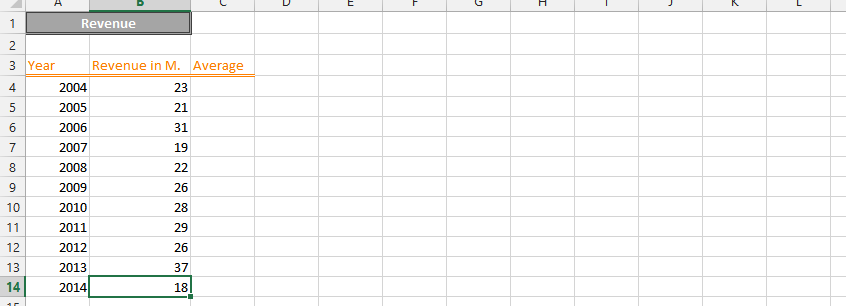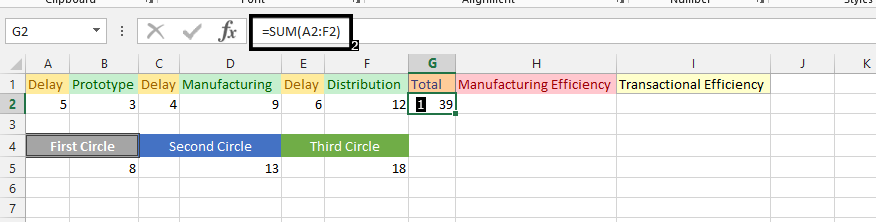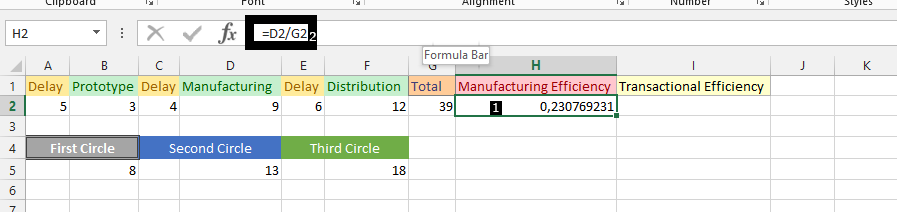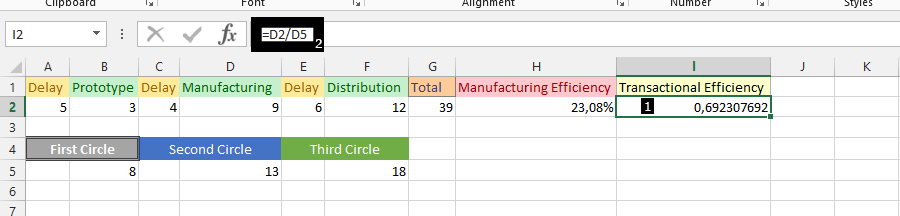#### How to Calculate Production Efficiency in Excel

Production efficiency is a measure of how effectively a company uses its resources to produce goods or services. It can be calculated in a number of ways, but one common method is to use Excel.

In this article, we will show you how to calculate production efficiency in Excel. We will also provide you with a free template that you can use to calculate production efficiency for your own business.

## Production data preparation

For the calculation of efficiency, we need data that looks like this:To calculate production efficiency in Excel, you will need the following data:

• The total time spent on the production process
• The amount of time that was actually productive

Once you have this data, you can use the following formula to calculate production efficiency:

``````Production Efficiency = (Actual Production Time / Total Production Time) * 100
``````

For example, if the total production time was 10 hours and the actual production time was 8 hours, then the production efficiency would be 80%.

Note: The scenario assumes knowledge of manufacturing efficiency and transactional efficiency. The numbers shown beneath the circles in the image above represent the sum of delays and processes (for example, Prototype).

## Efficiency calculations

Click under Total (G2) (1), and type =SUM(A2:F2).Click under Manufacturing Efficiency (H2) (1), and type =D2/G2 (2).Note: If you wish, you could right-click on the result, choose format cell, choose percentage, and then click ok. You can also use this step for other activities. The B2, D2, and F2 are Added-value time.

Click under transactional efficiency (I2) (1), and type =D2/D5 (second circle) (2).Note: You may need to adjust the formula based on your specific requirements and data. Also, make sure that the actual and standard output values are in the same units of measurement, such as units per hour or units per day, to ensure that the efficiency calculation is accurate.

In conclusion, it is about dividing the time that matters with total time to know how efficient it has been.

Calculating production efficiency in Excel is a simple process that can be used to measure the efficiency of your production process. By using this formula, you can identify areas where you can improve your efficiency and save time and money.

## Key points

• Production efficiency is a measure of how effectively a company uses its resources to produce goods or services.
• There are many ways to improve production efficiency, such as reducing waste, automating tasks, and using technology.
• The Toyota Production System (TPS) is a set of principles that can be used to improve production efficiency.
• By improving production efficiency, businesses can achieve a number of benefits, such as increased profits, improved customer service, and reduced environmental impact.